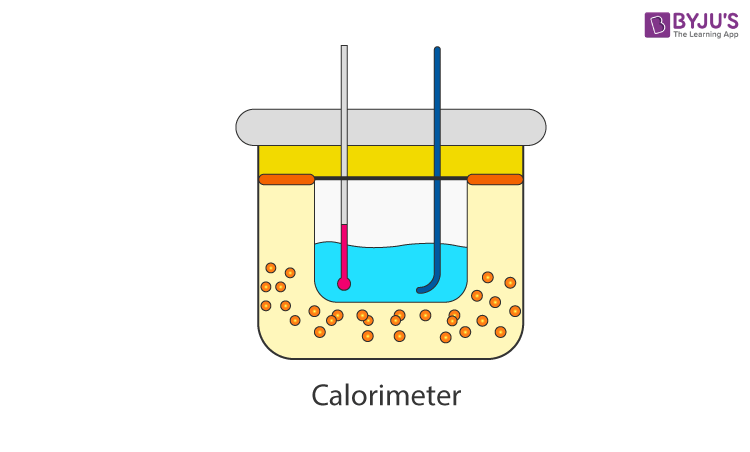# Principle Of Calorimetry

The Universe is made of matter and energy. The matter is made up of atoms and molecules, and energy makes these atoms and molecules to be in motion invariably – either by vibrating back and forth or by bumping into each other. This motion of molecules and atoms creates a form of energy that we know as the thermal energy or heat. Heat is present in all matter including the coldest voids of space. In this article, we discuss the method of measuring the heat transfer that occurs within a chemical reaction or other physical processes, and this method is known as the calorimetry.

## What is Calorimetry?

The act or science of measuring the changes in the state variables of a body in order to calculate the heat transfer associated with changes of its states such as physical changes or phase transitions under specific conditions is known as calorimetry. Calorimetry is performed with the help of a calorimeter.

## Calorimeter PrincipleWhen two bodies of different temperatures (preferably a solid and a liquid) are placed in physical contact with each other, the heat is transferred from the body with higher temperature to the body with lower temperature until thermal equilibrium is attained between them. The body at higher temperature releases heat while the body at lower temperature absorbs heat. The principle of calorimetry indicates the law of conservation energy, i.e. the total heat lost by the hot body is equal to the total heat gained by the cold body.

Heat Lost = Heat Gained

The heat transfer in a system is calculated using the formula,

$$q=mc\Delta t$$

Where

q is the measure of heat transfer

m is the mass of the body

c is the specific heat of the body

Δt is the change in the temperature

### Calorimeter Problems

Let us look at the example below to understand how to calculate the heat transfer between two objects.

Example 1.1: A metal weighing 4.82 g was heated to 115.0 °C and put into 35 mL of water of temperature 28.7 °C. The metal and water were allowed to come to an equilibrium temperature, determined to be 34.5 °C. Assuming no heat was lost to the environment, calculate the specific heat of the metal. Consider the specific heat capacity of water as 4.186 joule/gram °C.

Solution:

First, let us calculate the heat absorbed by the water and use the value obtained to calculate the specific heat of the metal.

The formula to find the heat absorbed by the water is given as

$$q=mc\Delta t$$

Substituting the values in the equation, we get

$$q_{absorbed}=(4.186\, J/g\cdot^{\circ}C)\times{35\,g}\times (5.8^{\circ}C)$$ $$q_{absorbed}=850J=q_{released}$$

Now, using this formula let us calculate the specific heat of the metal as follows:

$$850\,J=s\times 4.82\,g\times80.5^{\circ}C$$ $$s=2.19\, J/G\cdot ^{\circ}C$$

The specific heat of the metal is $$2.19\, J/G\cdot ^{\circ}C$$

The measurements obtained using the principle of calorimetry explain a lot of important phenomena in thermodynamics. Stay tuned to BYJU’S to learn more about calorimetry, heat transfer, and much more.